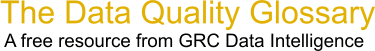Choose Index below for a list of all words and phrases defined in this glossary.

# Regression Equation

index | Index

## Regression Equation - definition(s)

regression equation - [statistics] The mathematical formula applied to independent variables to best predict the dependent variable being modeled. The notation in regression equations is always Y for the dependent variable and X for the independent variables. Each independent variable is associated with a regression coefficient describing the strength and sign of that variable's relationship to the dependent variable. A regression equation might look like this (where b represents a regression coefficient): Y = b0 + b1X1 + b2X2 + "¦bnXn

[Category=Geospatial ]

Source: esri, 30 July 2012 09:47:08, http://support.esri.com/en/knowledgebase/GISDictionary/term/abbreviationData Quality Glossary.  A free resource from GRC Data Intelligence. For comments, questions or feedback: dqglossary@grcdi.nl